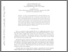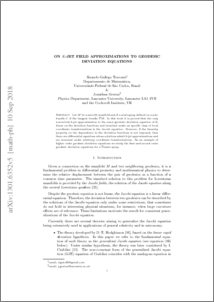# On K-Jet Field Approximations to Geodesic Deviation Equations

Gratus, Jonathan and Gallego Torrome, Ricardo (2018) On K-Jet Field Approximations to Geodesic Deviation Equations. International Journal of Geometric Methods in Modern Physics, 15 (12). ISSN 0219-8878Preview
PDF (1301.6352v5)
1301.6352v5.pdf - Accepted Version

## Abstract

Let M be a smooth manifold and S a semi-spray defined on a sub-bundle C of the tangent bundle TM . In this work it is proved that the only non-trivial k-jet approximation to the exact geodesic deviation equation of S, linear on the deviation functions and invariant under an specific class of local coordinate transformations is the Jacobi equation. However, if the linearity property on the dependence in the deviation functions is not imposed, then there are differential equations whose solutions admit k-jet approximations and are invariant under arbitrary coordinate transformations. As an example of higher order geodesic deviation equations we study the first and second order geodesic deviation equations for a Finsler spray.

Item Type:
Journal Article
Journal or Publication Title:
International Journal of Geometric Methods in Modern Physics
Uncontrolled Keywords:
/dk/atira/pure/subjectarea/asjc/3100/3101
Subjects:
Departments:
ID Code:
127450
Deposited By:
Deposited On:
12 Sep 2018 13:54
Refereed?:
Yes
Published?:
Published